• 求一个n个数的最大字段和问题，以及对其进行输出。基本的贪心算法问题。常用与研究生算法课程。
• 证明我就不够出了，我参考了这位博主的... 以下代码目的仅为记录分享，采用C++语言描述 腾讯出的题目是这样的： 一：递归描述： #include #include #include using namespace std; int max_csubstr(const string
证明我就不够出了，我参考了这位博主的博客 点击打开链接，以及这是麻省理工算法导论关于该问题的讲解视频 点击打开链接,我就是参看以上看明白的。

以下代码目的仅为记录和分享，采用C++语言描述

一：最大子序列

腾讯出的题目是这样的：1.递归描述：

#include <iostream>
#include <algorithm>
#include <string>
using namespace std;

int max_csubstr(const string &lhs, const string &rhs)
{
if(lhs.size() == 0 || rhs.size() == 0)
return 0;

if(lhs.back() == rhs.back())
return max_csubstr( lhs.substr(0, lhs.size()-1),
rhs.substr(0, rhs.size()-1) )+ 1;
else
return max( max_csubstr(lhs.substr(0, lhs.size()),
rhs.substr(0, rhs.size()-1)),
max_csubstr(lhs.substr(0, lhs.size()-1),
rhs.substr(0, rhs.size())) );
}

int main()
{
string sz;

while(cin >> sz){
int len = (int)sz.size();
if(len == 1)
cout << len << endl;
else{
string rev_sz(sz);
reverse(rev_sz.begin(), rev_sz.end());
cout << len - max_csubstr(sz, rev_sz) << endl;
}
}

return 0;
}
2.非递归描述：
#include <iostream>
#include <string>
#include <cstring>
#include <algorithm>
using namespace std;

#define MAX_SIZE 1001

int max_len[MAX_SIZE][MAX_SIZE];

int max_csubstr(const string &lhs, const string &rhs)
{
int len_lhs = (int)lhs.size();
int len_rhs = (int)rhs.size();

memset(max_len, 0, MAX_SIZE * MAX_SIZE);

for(int i=1; i<=len_lhs; ++i){
for(int j=1; j<=len_rhs; ++j){
if(lhs[i-1] == rhs[j-1])
max_len[i][j] = max_len[i-1][j-1] + 1;
else
max_len[i][j] = max(max_len[i-1][j], max_len[i][j-1]);
}
}

return max_len[len_lhs][len_rhs];
}

int main()
{
string sz;

while(cin >> sz){
int len = (int)sz.size();
if(len == 1)
cout << len << endl;
else{
string rev_sz(sz);
reverse(rev_sz.begin(), rev_sz.end());
cout << len - max_csubstr(sz, rev_sz) << endl;
}
}
return 0;
}
3.输出结果: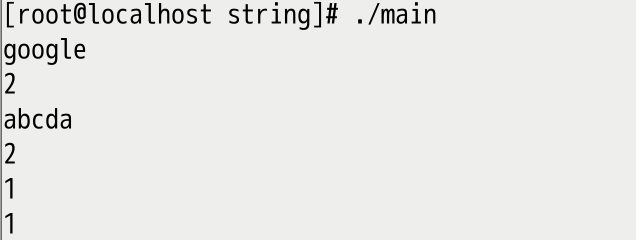二：最大字段和

问题描述：
给定由n个整数（包含负整数）组成的序列a1,a2,...,an，求该序列子段和的最大值。

当所有整数均为负值时定义其最大子段和为0。
依此定义，所求的最优值为：

例如，当(a1,a2 , a3 , a4 , a5 ,a6)=(-2,11,-4,13,-5,-2)时，

最大子段和为：

11+（-4）+13 =20

代码如下：

#include <iostream>
#include <cstring>
#include <vector>
using namespace std;

int find_max_add(vector<int> vec, const int size, int *start, int *end)
{
if(size == 0)
return -1;

int final_max = vec;
if(size != 1){
int cur_start = 0;
int cur_end = 0;

for(int i=0; i<size; ++i){
int cur_max = vec[i];
cur_start = i;
for(int j=i+1; j<size; ++j){
cur_max += vec[j];
if(cur_max > final_max){
final_max = cur_max;
*start = cur_start;
*end = j;
}
}
}
}
return final_max;
}

int main()
{
vector<int> vec;
int val;
while(cin >> val && val != -1){
vec.push_back(val);
}

int start = 0;
int end = 0;
int found = find_max_add(vec, vec.size(), &start, &end);
if(found != -1)
cout << start << "->" << end << ":" << found << endl;
else
cout << "input error." << endl;

return 0;
}

展开全文动态规划 最长子序列 递归 非递归 腾讯面试题
• 问题描述： 给定一个数字序列A1,A2,...An，求i,j(1<=i<=j<=n),使Ai+...Aj最大，输出这个最大。... 1，序列中有一个元素，就是A[i[自己，最大字段和是A[i]; 2，序列中有多个元素，以下标p...

问题描述：

给定一个数字序列A1,A2,...An，求i,j(1<=i<=j<=n),使Ai+...Aj最大，输出这个最大和。

设置一个数组dp[i]表示以A【i]作为末尾的连续序列的最大和，如果有n个数字，那么最大的字段和是dp,dp,...dp[n-1]中的最大值，现在求解dp数组。

因为dp[i]是以A[i]为结尾的连续序列，有以下两种情况，

1，序列中有一个元素，就是A[i[自己，最大字段和是A[i];

2，序列中有多个元素，以下标p开始，到A[i]结束，最大字段和dp[i-1]+A[i];

得到状态转移方程是

dp[i]=max{A[i],dp[i-1]+A[i]}

#include<cstdio>
#include<algorithm>
using namespace std;
const int maxn = 10010;
int A[maxn], dp[maxn];
int main() {
int n;
scanf("%d", &n);
for(int i=0;i<n;i++){
scanf("%d",&A[i]);
}
dp = 0;
for (int i = 1; i < n; i++)
dp[i] = max(A[i], dp[i - 1] + A[i]);
int k = 0;
for (int i = 1; i < n; i++) {
if (dp[i] > dp[k])
k = i;
}
printf("%d\n", dp[k]);
return 0;
}

展开全文算法 动态规划
• 求数组中满足给定的数对 给定两个有序整型数组ab，各有n个元素，求两个数组中满足给定的数对，即对a中元素ib中元素j，满足i + j = d(d已知) 分析 两个指针ij分别指向数组的首尾，然后从两端同时向...

求数组中满足给定和的数对

给定两个有序整型数组a和b，各有n个元素，求两个数组中满足给定和的数对，即对a中元素i和b中元素j，满足i + j = d(d已知)

分析

两个指针i和j分别指向数组的首尾，然后从两端同时向中间遍历，直到两个指针交叉。

代码

// 找出满足给定和的数对
void FixedSum(int* a, int* b, int n, int d)
{
for (int i = 0, j = n - 1; i < n && j >= 0)
{
if (a[i] + b[j] < d)
++i ;
else if (a[i] + b[j] == d)
{
cout << a[i] << ", " << b[j] << endl ;
++i ;
--j ;
}
else // a[i] + b[j] > d
--j ;
}
}

最大子段和

给定一个整型数组a，求出最大连续子段之和，如果和为负数，则按0计算，比如1， 2， -5， 6， 8则输出6 + 8 = 14

分析

编程珠玑上的经典题目，不多说了。

代码

// 子数组的最大和
int Sum(int* a, int n)
{
int curSum = 0;
int maxSum = 0;
for (int i = 0; i < n; i++)
{
if (curSum + a[i] < 0)
curSum = 0;
else
{
curSum += a[i] ;
maxSum = max(maxSum, curSum);
}
}
return maxSum;
}

最大子段积

给定一个整型数组a，求出最大连续子段的乘积，比如 1， 2， -8， 12， 7则输出12 * 7 = 84

分析

与最大子段和类似，注意处理负数的情况

代码

// 子数组的最大乘积
int MaxProduct(int *a, int n)
{
int maxProduct = 1; // max positive product at current position
int minProduct = 1; // min negative product at current position
int r = 1; // result, max multiplication totally

for (int i = 0; i < n; i++)
{
if (a[i] > 0)
{
maxProduct *= a[i];
minProduct = min(minProduct * a[i], 1);
}
else if (a[i] == 0)
{
maxProduct = 1;
minProduct = 1;
}
else // a[i] < 0
{
int temp = maxProduct;
maxProduct = max(minProduct * a[i], 1);
minProduct = temp * a[i];
}

r = max(r, maxProduct);
}

return r;
}
展开全文• 问题描述：给定由n个整数（可能有负整数）组成的序列（a1,a2,…,an），最大子段问题要求该序列形如 的最大值（1<=i<=j<=n），当序列中所有整数均为负整数时，其最大子段为0。 1.简单算法 代码如下： ...

问题描述：给定由n个整数（可能有负整数）组成的序列（a1,a2,…,an），最大子段和问题要求该序列形如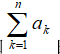的最大值（1<=i<=j<=n），当序列中所有整数均为负整数时，其最大子段和为0。

1.简单算法
代码如下：

#include<stdio.h>
#include<stdlib.h>

#define max 6

int MUL(int *p)
{
int sum = 0, m = 0;

for (int i = 0; i < max; i++)
{
if (p[i] > 0)//定位到序列中第一个正数
{
for (; i < max; i++)//从第一个正数开始
{
sum += p[i];//从第一个整数开始往后求和
if (m < sum)
m = sum;//m用来保存期间出现的最大和，即所求
}
break;//跳出循环，主要是跳出第一个for;他的作用只是定位到第一个，目的达到没必要进行
}
}
return m;
}

int main()
{
int a[max];//用来存储序列
printf("请输入序列：");
for (int i = 0; i < max; i++)
scanf_s("%d", &a[i]);//vs中使用scanf_s,vc中scanf
printf("最大字段和为：%d\n", MUL(a));
system("PAUSE");
return 0;
}

示例输入输出：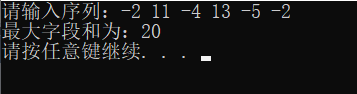2.分治法
分治法的思想就是将完整的序列一分为二（下面称左段右段），分别求两段子序列的最大字段和来求解。最后结果会有三种情况
（1）整个序列的最大子字段（s）与左段最大子字段（s1）相同
（2）整个序列的最大子字段(s)与右段最大子字段（s2）相同
（3）整个序列的最大子字段(s)恰好横跨左右段，即左右段的最大子字段是整个序列最大子字段的子字段(s=s1+s2)
代码如下：

#include<stdio.h>
#include<stdlib.h>

#define max 6
int MaxSubSum(int *a, int left, int right)//参数为数组a，left表示数组左下标，right表示数组右下标
{
int sum = 0;
if (left == right)//序列长度为一的情况
sum = a[left] > 0 ? a[left] : 0;
else
{
int center = (left + right) / 2;//center为数组中间下标
int leftsum = MaxSubSum(a, left, center);//求leftsum数组左段和
int rightsum = MaxSubSum(a, center + 1, right);//求rightsum数组右段和

int s1 = 0;//记录左段最大字段和
int lefts = 0;
for (int i = center; i >= left; i--)
{
lefts += a[i];
if (lefts > s1)
s1 = lefts;
}

int s2 = 0;//记录右段最大字段和
int rights = 0;
for (int i = center + 1; i <= right; i++)
{
rights += a[i];
if (rights > s2)
s2 = rights;
}
//分治法三种情况比较，确定整个序列最大字段和
sum = s1 + s2;
if (sum < leftsum)
sum = leftsum;
if (sum < rightsum)
sum = rightsum;
}
return sum;
}
//辅助调用，可替掉
int MaxSum(int n, int *a)
{
return MaxSubSum(a, 1,n);
}
int main()
{
int a[max];
printf("请输入序列：");
for (int i = 1; i <= max; i++)
scanf_s("%d", &a[i]);
printf("最大字段和为：%d\n", MaxSum(max, a));
system("PAUSE");
return 0;
}

示例输入输出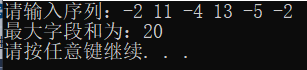3.动态规划
代码如下：

#include<stdio.h>
#include<stdlib.h>
#define MAX 6
//本例为最大m子段和问题解法，最大子段和为本例中m=1时的特殊情况
int MaxSum(int m, int n, int *a)
{
if (n < m || m < 1)
return 0;
int *b = new int[n + 1];
int *c = new int[n + 1];
b = 0;
c = 0;
for (int i = 1; i <= m; i++)
{
b[i] = b[i - 1] + a[i];
c[i - 1] = b[i];
int max = b[i];
for (int j = i + 1; j <= i + n - m; j++)
{
b[j] = b[j - 1] > c[j - 1] ? b[j - 1] + a[j] : c[j - 1] + a[j];
c[j - 1] = max;
if (max < b[j])
max = b[j];
}
c[i + n - m] = max;
}
int sum = 0;
for (int j = m; j <= n; j++)
if (sum < b[j])
sum = b[j];
return sum;
}
int main()
{
int a[MAX];
printf("请输入序列：");
for (int i = 1; i <= MAX; i++)
{
scanf_s("%d", &a[i]);
}
printf("最大子字段和为：%d\n", MaxSum(1, MAX, a));
system("PAUSE");
return 0;
}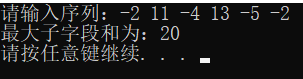参考算法与设计第五版王晓东
为表述清楚，增加了许多不必要代码，可自行优化
学习中，欢迎交流，指导

展开全文• 最大字段和

2014-04-17 17:48:09
• 最大子段和及其下标（分治算法） 这个是王晓东《计算机算法设计与分析》第58页到59页最大子段和分治算法改写成除计算最大子段和外还要记录最大字段和的起点与终点。最大字段和分治算法
• 动态规划（Dynamic Programming, DP）为一常用算法思想，本文讲述如何利用DP解决常见的最大字段和及其变种问题。一、 最大字段和问题问题定义设数组为a[k]a[k]，1≤k≤n1 \le k \le n，最大字段和XX定义为：X=max1≤...动态规划 最大字段和 DP
• 序列(-20,11,-4,13,-5,-12)动态规划法求解最大子段问题的关键是要确定动态规划函数。 aj的j为下标 b(j)=b(j-1)+aj b(j-1)>0 b(j)=aj b(j-1) #include using namespace std; #define M 100 int maxadd...动态规划 算法
• 给定n个数组成的序列，求其中最大子段和，并规定其中如果所有数均为负值的时候，那么最大字段和为零。 解决这样的问题需要用的算法是：分治法 基本思路： 1. 划分两个长度基本相同的子段，得出以下三种情况 2. 如果...C++ 算法 最大字段和 分治法
• 最大连续字段和

千次阅读 2016-12-27 20:22:27
最大子段 输入 有多组测试数据。 每一组的第一行是一个整数nn。 下面一行是nn个以空格分开的整数...对于每一组数据，输出最大子段，占一行。 样例输入 6 -1 4 -1 -5 5 1 样例输出 9 思路：遇到负数停一
• 表：t_test -------------------------------------- id(int) cost(int) des Autoid(id) -------------------------------------- 1 10 aaaa 1 1 15 bbbb 2 1 20 cccc 3 ...取每一类id中cost最大的纪录
• * 一维最大字段和问题，约定如果全部元素均是负数， * 则最大字段和为0（不选任何元素）。 * @author Sking */ package 数组问题; public class 一维最大子段和 { /** * 暴力遍历求解最大字段和问题 * ...java Java JAVA 数组 最大字段和
• //输入一组整数，求出这组数字子序列最大值 #include int MAxSum(int arr[],int len) { int maxsum = 0; int i; int j; for (i = 0; i ; i++) { int thissum = 0; for (j = i; j ; j++) { ...
• 排序 按某个字段进行排序（默认是升序）： select name,birth from pet order by birth; 按某个字段进行降序排序： ...求上面这张表的article字段最大值： select max(article) as max from shop; ...
•  虽然hive中有minmax，但是那是求某列字段的最小值和最大值，在这里行不通。接下来使用hive中的数组排序方法来求解。  思路： 先将字段合并到数组里面，然后使用数组排序函数。 select sort_array(array...
• select *  from (select t.*, row_number() over (order by worklist_id desc) as rnum  from table_name t where t.wf_serial_no='1342121')   where rnum = 1;Oracle
• SELECT t2.* FROM ( SELECT 分组字段, max(最大字段) 最大字段 FROM 表名 GROUP BY 分组字段 ) t1 INNER JOIN 表名 t2 ON t1.分组字段=t2.分组字段 AND t1.最大字段 = t2.最大字段 GROUP BY t1.分组字段 ...mysql
• 我遇到的问题是工商数据id bignumber 16 太长，1是表输入时报错 无法转换，2是文本文件输出时报错 arraysize exceeds VM. 第一个问题 表输入报错 解决：cast(table.id as String) 第二个问题 报 java.lang....spark hadoop
• 最大子段问题的动态规划求解最大子段问题的动态规划求解最大子段问题的动态规划求解最大子段问题的动态规划求解acm
• 先把最大品类的字段添加到最后,作为值出现(sql中不能直接拿到字段名) 在查询最大值对应的字段名 添加最大值对应的字段名到值中 select shop, month, dz, fz, sp, case when dz>fz and dz>sp then '...
• 二、分组统计并求最大时间 db.sendlog.group({ key:{template:"$template",event:"$event",channel:"\$channel"},//根据mongodb中的字段分组 initial: { fai...
• 带输入输出界面的最大子段问题 用java编写的
• greatest(字段1，字段2，字段3，..，字段n) 取最大值 least(字段1，字段2，字段3，...，字段n) 取最小值 示例： SELECT GREATEST(DATE('2016-05-02'), DATE('2015-05-02'), DATE('2017-05-02')) from DUAL; ##结果...
• 算法分析中的一个典型问题2.10最大字段问题，给出一组数，求相连的几个数的最大值如： 输入: 6 -5 8 -1 -9 6 -2 输出： 8 即：8+（-1）+（-9）+6 = 8
• 利用动态规划法求一个数组最大的子段，并输出最大字段（JAVA实现）...